# Cosmetics

## 89 products

1.\$7.90

2.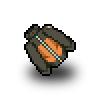\$7.90

3.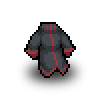\$7.90

4.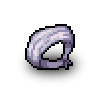\$4.90

5.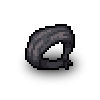\$4.90

6.\$4.90

7.\$4.90

8.\$4.90

9.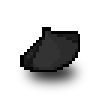\$4.90

10.\$4.90

11.\$4.90

12.\$4.90

13.\$4.90

14.\$4.90

15.\$4.90

16.\$4.90

17.\$4.90

18.\$9.90

19.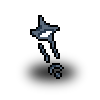\$4.90

20.\$4.90

21.\$4.90

22.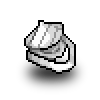\$9.90

23.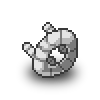\$9.90

24.\$9.90

25.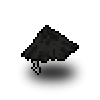\$9.90

26.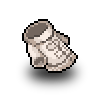\$7.90

27.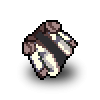\$9.90

28.\$9.90

29.\$9.90

30.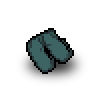\$4.90

31.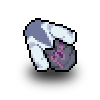\$9.90

32.\$7.90

33.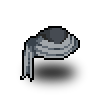\$7.90

34.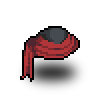\$7.90

35.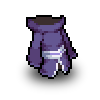\$9.90

36.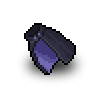\$9.90

37.\$9.90

38.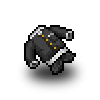\$9.90

39.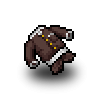\$9.90

40.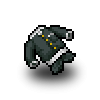\$9.90

41.\$9.90

42.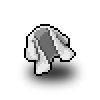\$7.90

43.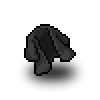\$7.90

44.\$7.90

45.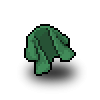\$7.90

46.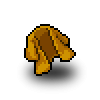\$7.90

47.\$7.90

48.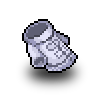\$7.90

49.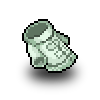\$7.90

50.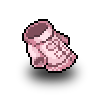\$7.90